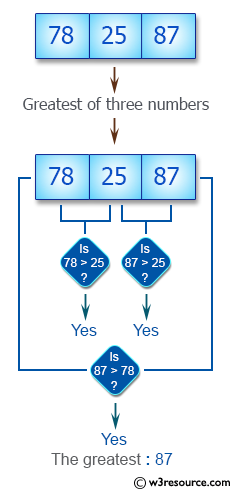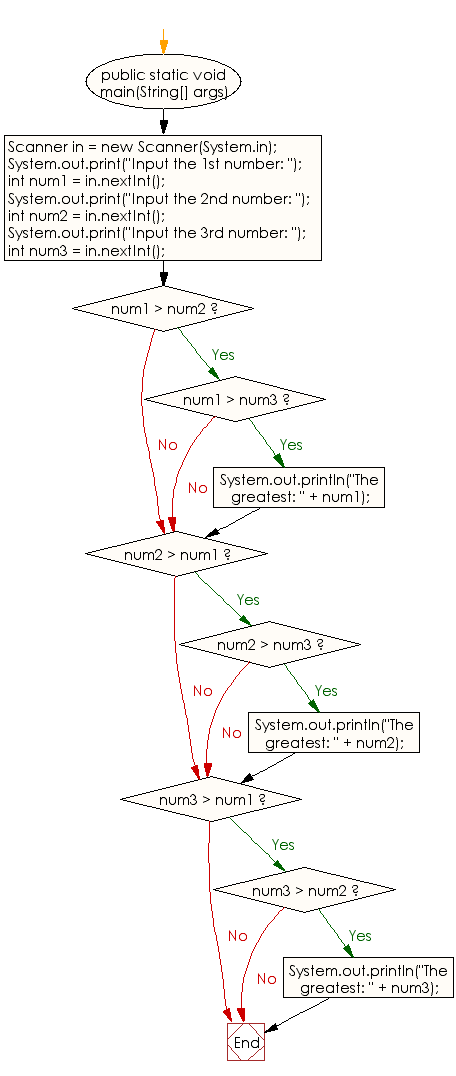﻿ Java exercises: Find the greatest of three numbers - w3resource# Java Conditional Statement Exercises: Find the greatest of three numbers

## Java Conditional Statement: Exercise-3 with Solution

Take three numbers from the user and print the greatest number.

Test Data
Input the 1st number: 25
Input the 2nd number: 78
Input the 3rd number: 87

Pictorial Presentation:Sample Solution:

Java Code:

``````import java.util.Scanner;
public class Exercise3 {

public static void main(String[] args) {
Scanner in = new Scanner(System.in);

System.out.print("Input the 1st number: ");
int num1 = in.nextInt();

System.out.print("Input the 2nd number: ");
int num2 = in.nextInt();

System.out.print("Input the 3rd number: ");
int num3 = in.nextInt();

if (num1 > num2)
if (num1 > num3)
System.out.println("The greatest: " + num1);

if (num2 > num1)
if (num2 > num3)
System.out.println("The greatest: " + num2);

if (num3 > num1)
if (num3 > num2)
System.out.println("The greatest: " + num3);
}
}
```
```

Sample Output:

```Input the 1st number: 25
Input the 2nd number: 78
Input the 3rd number: 87
The greatest: 87
```

Flowchart:Java Code Editor:

What is the difficulty level of this exercise?

﻿

## Java: Tips of the Day

Cast Double to Integer in Java:

A Double is not an Integer, so the cast won't work. Note the difference between the Double class and the double primitive. Also note that a Double is a Number, so it has the method intValue, which you can use to get the value as a primitive int.

Ref: https://bit.ly/3vjEae5